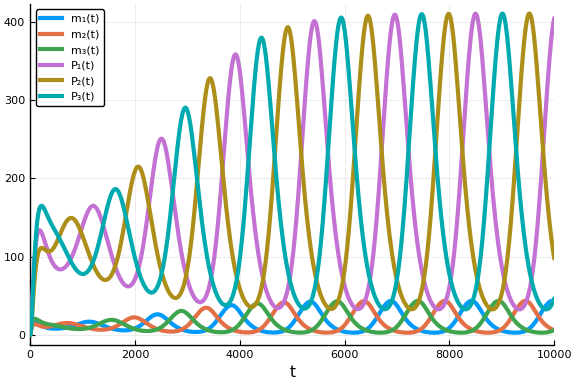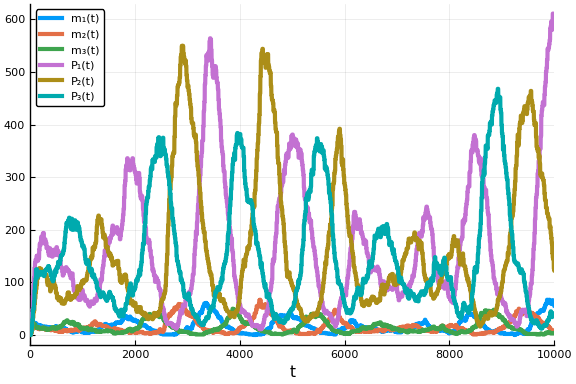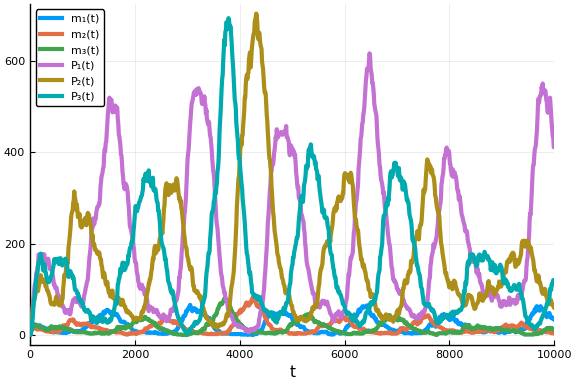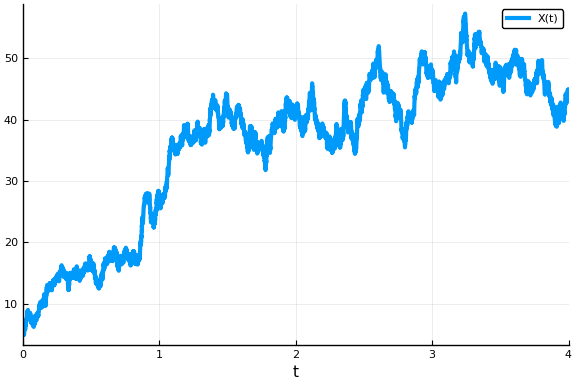DiffEqBiological Tutorial I: Introduction

Samuel Isaacson

DiffEqBiological.jl is a domain specific language (DSL) for writing chemical reaction networks in Julia. The generated chemical reaction network model can then be translated into a variety of mathematical models which can be solved using components of the broader DifferentialEquations.jl ecosystem.

In this tutorial we'll provide an introduction to using DiffEqBiological to specify chemical reaction networks, and then to solve ODE, jump, tau-leaping and SDE models generated from them. Let's start by using the DiffEqBiological reaction_network macro to specify a simply chemical reaction network; the well-known Repressilator.

We first import the basic packages we'll need, and use Plots.jl for making figures:

# If not already installed, first hit "]" within a Julia REPL. Then type:
# add DifferentialEquations DiffEqBiological PyPlot Plots Latexify

using DifferentialEquations, DiffEqBiological, Plots, Latexify
pyplot(fmt=:svg);

We now construct the reaction network. The basic types of arrows and predefined rate laws one can use are discussed in detail within the DiffEqBiological Chemical Reaction Models documentation. Here we use a mix of first order, zero order and repressive Hill function rate laws. Note, $\varnothing$ corresponds to the empty state, and is used for zeroth order production and first order degradation reactions:

repressilator = @reaction_network begin
hillr(P₃,α,K,n),  --> m₁
hillr(P₁,α,K,n),  --> m₂
hillr(P₂,α,K,n),  --> m₃
(δ,γ), m₁
(δ,γ), m₂
(δ,γ), m₃
β, m₁ --> m₁ + P₁
β, m₂ --> m₂ + P₂
β, m₃ --> m₃ + P₃
μ, P₁ -->
μ, P₂ -->
μ, P₃ -->
end α K n δ γ β μ;

We can use Latexify to look at the corresponding reactions and understand the generated rate laws for each reaction

latexify(repressilator; env=:chemical)
\begin{align*} \require{mhchem} \ce{ \varnothing &->[\frac{\alpha \cdot K^{n}}{K^{n} + P_3^{n}}] m_{1}}\\ \ce{ \varnothing &->[\frac{\alpha \cdot K^{n}}{K^{n} + P_1^{n}}] m_{2}}\\ \ce{ \varnothing &->[\frac{\alpha \cdot K^{n}}{K^{n} + P_2^{n}}] m_{3}}\\ \ce{ m_{1} &<=>[\delta][\gamma] \varnothing}\\ \ce{ m_{2} &<=>[\delta][\gamma] \varnothing}\\ \ce{ m_{3} &<=>[\delta][\gamma] \varnothing}\\ \ce{ m_{1} &->[\beta] m_{1} + P_{1}}\\ \ce{ m_{2} &->[\beta] m_{2} + P_{2}}\\ \ce{ m_{3} &->[\beta] m_{3} + P_{3}}\\ \ce{ P_{1} &->[\mu] \varnothing}\\ \ce{ P_{2} &->[\mu] \varnothing}\\ \ce{ P_{3} &->[\mu] \varnothing} \end{align*}

We can also use Latexify to look at the corresponding ODE model for the chemical system

latexify(repressilator)
\begin{align*} \frac{dm_1}{dt} =& \frac{\alpha \cdot K^{n}}{K^{n} + P_3^{n}} - \delta \cdot m_1 + \gamma \\ \frac{dm_2}{dt} =& \frac{\alpha \cdot K^{n}}{K^{n} + P_1^{n}} - \delta \cdot m_2 + \gamma \\ \frac{dm_3}{dt} =& \frac{\alpha \cdot K^{n}}{K^{n} + P_2^{n}} - \delta \cdot m_3 + \gamma \\ \frac{dP_1}{dt} =& \beta \cdot m_1 - \mu \cdot P_1 \\ \frac{dP_2}{dt} =& \beta \cdot m_2 - \mu \cdot P_2 \\ \frac{dP_3}{dt} =& \beta \cdot m_3 - \mu \cdot P_3 \\ \end{align*}

To solve the ODEs we need to specify the values of the parameters in the model, the initial condition, and the time interval to solve the model on. To do this it helps to know the orderings of the parameters and the species. Parameters are ordered in the same order they appear after the end statement in the @reaction_network macro. Species are ordered in the order they first appear within the @reaction_network macro. We can see these orderings using the speciesmap and paramsmap functions:

speciesmap(repressilator)
OrderedCollections.OrderedDict{Symbol,Int64} with 6 entries:
:m₁ => 1
:m₂ => 2
:m₃ => 3
:P₁ => 4
:P₂ => 5
:P₃ => 6
paramsmap(repressilator)
OrderedCollections.OrderedDict{Symbol,Int64} with 7 entries:
:α => 1
:K => 2
:n => 3
:δ => 4
:γ => 5
:β => 6
:μ => 7

Solving the ODEs:

Knowing these orderings, we can create parameter and initial condition vectors, and setup the ODEProblem we want to solve:

# parameters [α,K,n,δ,γ,β,μ]
p = (.5, 40, 2, log(2)/120, 5e-3, 20*log(2)/120, log(2)/60)

# initial condition [m₁,m₂,m₃,P₁,P₂,P₃]
u₀ = [0.,0.,0.,20.,0.,0.]

# time interval to solve on
tspan = (0., 10000.)

# create the ODEProblem we want to solve
oprob = ODEProblem(repressilator, u₀, tspan, p)
ODEProblem with uType Array{Float64,1} and tType Float64. In-place: true
timespan: (0.0, 10000.0)
u0: [0.0, 0.0, 0.0, 20.0, 0.0, 0.0]

At this point we are all set to solve the ODEs. We can now use any ODE solver from within the DiffEq package. We'll just use the default DifferentialEquations solver for now, and then plot the solutions:

sol = solve(oprob, saveat=10.)
plot(sol, fmt=:svg)We see the well-known oscillatory behavior of the repressilator! For more on choices of ODE solvers, see the JuliaDiffEq documentation.

Stochastic Simulation Algorithms (SSAs) for Stochastic Chemical Kinetics

Let's now look at a stochastic chemical kinetics model of the repressilator, modeling it with jump processes. Here we will construct a DiffEqJump JumpProblem that uses Gillespie's Direct method, and then solve it to generate one realization of the jump process:

# first we redefine the initial condition to be integer valued
u₀ = [0,0,0,20,0,0]

# next we create a discrete problem to encode that our species are integer valued:
dprob = DiscreteProblem(repressilator, u₀, tspan, p)

# now we create a JumpProblem, and specify Gillespie's Direct Method as the solver:
jprob = JumpProblem(dprob, Direct(), repressilator, save_positions=(false,false))

# now let's solve and plot the jump process:
sol = solve(jprob, SSAStepper(), saveat=10.)
plot(sol, fmt=:svg)Here we see that oscillations remain, but become much noiser. Note, in constructing the JumpProblem we could have used any of the SSAs that are part of DiffEqJump instead of the Direct method, see the list of SSAs (i.e. constant rate jump aggregators) in the documentation.

$\tau$-leaping Methods:

While SSAs generate exact realizations for stochastic chemical kinetics jump process models, $\tau$-leaping methods offer a performant alternative by discretizing in time the underlying time-change representation of the stochastic process. The DiffEqJump package has limited support for $\tau$-leaping methods in the form of the basic Euler's method type approximation proposed by Gillespie. We can simulate a $\tau$-leap approximation to the repressilator by using the RegularJump representation of the network to construct a JumpProblem:

rjs = regularjumps(repressilator)
lprob = JumpProblem(dprob, Direct(), rjs)
lsol = solve(lprob, SimpleTauLeaping(), dt=.1)
plot(lsol, plotdensity=1000, fmt=:svg)Chemical Langevin Equation (CLE) Stochastic Differential Equation (SDE) Models:

At an intermediary physical scale between macroscopic ODE models and microscopic stochastic chemical kinetic models lies the CLE, a SDE version of the model. The SDEs add to each ODE above a noise term. As the repressilator has species that get very close to zero in size, it is not a good candidate to model with the CLE (where solutions can then go negative and become unphysical). Let's create a simpler reaction network for a birth-death process that will stay non-negative:

bdp = @reaction_network begin
c₁, X --> 2X
c₂, X --> 0
c₃, 0 --> X
end c₁ c₂ c₃
p = (1.0,2.0,50.)
u₀ = [5.]
tspan = (0.,4.);

The corresponding Chemical Langevin Equation SDE is then

$dX_t = \left(c_1 X - c_2 X + c_3 \right) dt + \left( \sqrt{c_1 X} - \sqrt{c_2 X} + \sqrt{c_3} \right)dW_t,$

where $W_t$ denotes a standard Brownian Motion. We can solve the CLE SDE model by creating an SDEProblem and solving it similar to what we did for ODEs above:

# SDEProblem for CLE
sprob = SDEProblem(bdp, u₀, tspan, p)

# solve and plot, tstops is used to specify enough points
# that the plot looks well-resolved
sol = solve(sprob, tstops=range(0., step=4e-3, length=1001))
plot(sol, fmt=:svg)We again have complete freedom to select any of the StochasticDifferentialEquations.jl SDE solvers, see the documentation.

What information can be queried from the reaction_network:

The generated reaction_network contains a lot of basic information. For example

• f=oderhsfun(repressilator) is a function f(du,u,p,t) that given the current state vector u and time t fills du with the time derivatives of u (i.e. the right hand side of the ODEs).

• jac=jacfun(repressilator) is a function jac(J,u,p,t) that evaluates and returns the Jacobian of the ODEs in J. A corresponding Jacobian matrix of expressions can be accessed using the jacobianexprs function:

latexify(jacobianexprs(repressilator))
\begin{equation*} \left[ \begin{array}{cccccc} - \delta & 0 & 0 & 0 & 0 & \frac{ - K^{n} \cdot n \cdot \alpha \cdot P_3^{-1 + n}}{\left( K^{n} + P_3^{n} \right)^{2}} \\ 0 & - \delta & 0 & \frac{ - K^{n} \cdot n \cdot \alpha \cdot P_1^{-1 + n}}{\left( K^{n} + P_1^{n} \right)^{2}} & 0 & 0 \\ 0 & 0 & - \delta & 0 & \frac{ - K^{n} \cdot n \cdot \alpha \cdot P_2^{-1 + n}}{\left( K^{n} + P_2^{n} \right)^{2}} & 0 \\ \beta & 0 & 0 & - \mu & 0 & 0 \\ 0 & \beta & 0 & 0 & - \mu & 0 \\ 0 & 0 & \beta & 0 & 0 & - \mu \\ \end{array} \right] \end{equation*}
• pjac = paramjacfun(repressilator) is a function pjac(pJ,u,p,t) that evaluates and returns the Jacobian, pJ, of the ODEs with respect to the parameters. This allows reaction_networks to be used in the DifferentialEquations.jl local sensitivity analysis package DiffEqSensitivity.

By default, generated ODEProblems will be passed the corresponding Jacobian function, which will then be used within implicit ODE/SDE methods.

The DiffEqBiological API documentation provides a thorough description of the many query functions that are provided to access network properties and generated functions. In DiffEqBiological Tutorial II we'll explore the API.

Getting Help

Have a question related to DiffEqBiological or this tutorial? Feel free to ask in the DifferentialEquations.jl Gitter. If you think you've found a bug in DiffEqBiological, or would like to request/discuss new functionality, feel free to open an issue on Github (but please check there is no related issue already open). If you've found a bug in this tutorial, or have a suggestion, feel free to open an issue on the DiffEqTutorials Github site. Or, submit a pull request to DiffEqTutorials updating the tutorial!

Appendix

This tutorial is part of the DiffEqTutorials.jl repository, found at: https://github.com/JuliaDiffEq/DiffEqTutorials.jl

To locally run this tutorial, do the following commands:

using DiffEqTutorials
DiffEqTutorials.weave_file("models","03-diffeqbio_I_introduction.jmd")

Computer Information:

Julia Version 1.1.1
Commit 55e36cc308 (2019-05-16 04:10 UTC)
Platform Info:
OS: Linux (x86_64-pc-linux-gnu)
CPU: Intel(R) Core(TM) i7-3770 CPU @ 3.40GHz
WORD_SIZE: 64
LIBM: libopenlibm
LLVM: libLLVM-6.0.1 (ORCJIT, ivybridge)

Package Information:

Status ~/.julia/environments/v1.1/Project.toml
[7e558dbc-694d-5a72-987c-6f4ebed21442] ArbNumerics 0.5.4
[6e4b80f9-dd63-53aa-95a3-0cdb28fa8baf] BenchmarkTools 0.4.2
[be33ccc6-a3ff-5ff2-a52e-74243cff1e17] CUDAnative 2.2.0
[3a865a2d-5b23-5a0f-bc46-62713ec82fae] CuArrays 1.0.2
[55939f99-70c6-5e9b-8bb0-5071ed7d61fd] DecFP 0.4.8
[abce61dc-4473-55a0-ba07-351d65e31d42] Decimals 0.4.0
[ebbdde9d-f333-5424-9be2-dbf1e9acfb5e] DiffEqBayes 1.1.0
[eb300fae-53e8-50a0-950c-e21f52c2b7e0] DiffEqBiological 3.8.2
[459566f4-90b8-5000-8ac3-15dfb0a30def] DiffEqCallbacks 2.5.2
[f3b72e0c-5b89-59e1-b016-84e28bfd966d] DiffEqDevTools 2.9.0
[1130ab10-4a5a-5621-a13d-e4788d82bd4c] DiffEqParamEstim 1.6.0
[055956cb-9e8b-5191-98cc-73ae4a59e68a] DiffEqPhysics 3.1.0
[6d1b261a-3be8-11e9-3f2f-0b112a9a8436] DiffEqTutorials 0.1.0
[0c46a032-eb83-5123-abaf-570d42b7fbaa] DifferentialEquations 6.4.0
[31c24e10-a181-5473-b8eb-7969acd0382f] Distributions 0.20.0
[497a8b3b-efae-58df-a0af-a86822472b78] DoubleFloats 0.9.1
[f6369f11-7733-5829-9624-2563aa707210] ForwardDiff 0.10.3
[4138dd39-2aa7-5051-a626-17a0bb65d9c8] JLD 0.9.1
[23fbe1c1-3f47-55db-b15f-69d7ec21a316] Latexify 0.8.2
[eff96d63-e80a-5855-80a2-b1b0885c5ab7] Measurements 2.0.0
[961ee093-0014-501f-94e3-6117800e7a78] ModelingToolkit 0.2.0
[76087f3c-5699-56af-9a33-bf431cd00edd] NLopt 0.5.1
[2774e3e8-f4cf-5e23-947b-6d7e65073b56] NLsolve 4.0.0
[429524aa-4258-5aef-a3af-852621145aeb] Optim 0.18.1
[1dea7af3-3e70-54e6-95c3-0bf5283fa5ed] OrdinaryDiffEq 5.8.1
[65888b18-ceab-5e60-b2b9-181511a3b968] ParameterizedFunctions 4.1.1
[91a5bcdd-55d7-5caf-9e0b-520d859cae80] Plots 0.25.1
[d330b81b-6aea-500a-939a-2ce795aea3ee] PyPlot 2.8.1
[731186ca-8d62-57ce-b412-fbd966d074cd] RecursiveArrayTools 0.20.0
[90137ffa-7385-5640-81b9-e52037218182] StaticArrays 0.11.0
[f3b207a7-027a-5e70-b257-86293d7955fd] StatsPlots 0.11.0